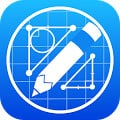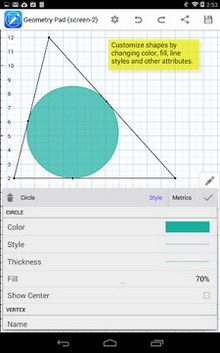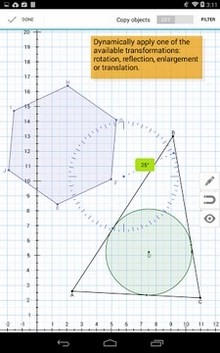Geometry Pad is a dynamic geometry application for Android tablets with universal appeal. Teachers can use it in a geometry class for better students engagement and deeper understanding of geometric concepts. Students would benefit from using Geometry Pad while working on geometry assignments at home as well. Easily create complex geometric sketches, measure everything you have in your document, experiment with shapes and transformations.
Use following tools to sketch your geometry constructions (*):
– Move and scale. Scroll the workbook by your finger. Pinch and zoom the content of your workbook. Move and modify geometric shapes.
– Compass to create arcs.
– Point. Plot a point on the workbook. Customize point name and color.
– Line. Create a line. Customize line style, color and start/end points. Calculate line length (distance between points).
– Midpoint for lines. Show/hide midpoint for lines and polygon sides. Snap to midpoints.
– Parallel, perpendicular and tangent lines. The lines can be created as easily as regular lines. Just create and move the line until it automatically snaps to parallel, perpendicular or tangent.
– Angle. Create an angle with up to 1 degree precision. Customize angle line style and color.
– Triangle. Create a triangle of one of the predefined types: regular, right triangle, isosceles, equilateral. Customize sides style and color. Calculate triangle perimeter and area. Calculate inner angles of the triangle and length of its sides.
– Triangle lines. Create altitude, bisector and median lines in a triangle. Calculate length of triangle lines. Customize lines style and color.
– Quadrilateral. Create a quadrilateral of one of the predefined types: regular, square, rectangle, parallelogram, rhombus. Customize sides style and color. Calculate quadrilateral perimeter and area. Calculate inner angles of the quadrilateral and length of its sides.
– Circle. Create a circle. Calculate circle perimeter and area. Easily create circles inscribed into a triangle by placing circle’s center close enough to the incenter of a triangle.
– Circle radius and chord. Create radius and chord lines for a circle. Customize lines style and color. Calculate the length of the lines.
– Polygons and regular polygons.
– Arcs and circular sectors.
– Ellipses.
– Text annotations. Create floating and pinned single and multiple lines text annotations. Customize text/background color and transparency.
– Measurements tool. Measure shape properties in single touch. Measure intersection points (line & line, line & circle). Use multitouch to measure distance between points and angle between lines.
– Transformation tools: rotation, reflection, enlargement and translation.
– Manual input of coordinates, lengths and angles. Use manual input to precisely locate points, setup custom length for a line or a polygon side, and change angle value.
– Built-in calculator: basic arithmetic functions, square root, sin, cos, tan.
Manage multiple documents with your geometric sketches at the same time using Save and Load features. In addition, you can share documents through e-mail or Dropbox.